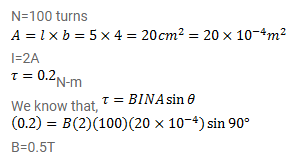# Question 53 A rectangular coil of 100 turns has length 5cm and width 4cm.

Question:

A rectangular coil of 100 turns has length $5 \mathrm{~cm}$ and width $4 \mathrm{~cm}$. It is placed with its plane parallel to a uniform magnetic field and a current of $2 \mathrm{~A}$ is sent through the coil. Find the magnitude of the magnetic field $\mathrm{B}$, if the torque acting on the coil is $0.2 \mathrm{~N}-\mathrm{m}$.

Solution: